#Function Repository Resource:

# GeneralMiniMaxApproximation

Find a mini-max approximation of a function defined parametrically

Contributed by: Wolfram Research
 ResourceFunction["GeneralMiniMaxApproximation"][{fx,fy},{t,{t0,t1},m,n},x] finds the rational polynomial function of x, with numerator order m and denominator order n, that gives a mini-max approximation to the curve with x and y coordinates fx and fy generated as a function of t on the interval t0 to t1. ResourceFunction["GeneralMiniMaxApproximation"][{fx,fy},approx,{t,{t0,t1},m,n},x] finds the mini-max approximation, starting the iterative algorithm with approx.

## Details and Options

ResourceFunction["GeneralMiniMaxApproximation"] by default minimizes the maximum value of the relative error between the approximation and expr.
ResourceFunction["GeneralMiniMaxApproximation"][{fx,fy,g},{t,{t0,t1},m,n},x] computes the error using a factor of g. In this case, the mini-max approximation returned by ResourceFunction["GeneralMiniMaxApproximation"] is the rational function h[x] that minimizes the maximum value of the quantity (fy-h[fx])/g.
ResourceFunction["GeneralMiniMaxApproximation"] returns {abscissa,{approximation,maxerror}}, where abscissa is a list of the abscissas where the relative error is a local maximum, approximation is the rational approximant, and maxerror is the global maximum of the relative error.
When an approximation is given as the second argument of ResourceFunction["GeneralMiniMaxApproximation"], it must have the same form as the result returned by ResourceFunction["GeneralMiniMaxApproximation"].
ResourceFunction["GeneralMiniMaxApproximation"] takes the same options as MiniMaxApproximation.
In the "Derivatives" option, a list of derivatives must give derivatives for both parts of the parametrically defined function.

## Examples

### Basic Examples

A degree (2,4) mini-max approximation to the inverse of Exp:

 In:=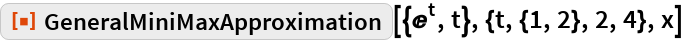Out=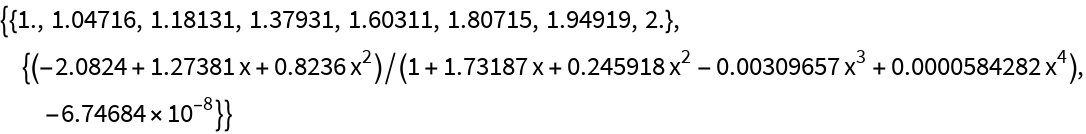Extract the approximation and plot the relative error in the approximation over the interval e≤x≤e2:

 In:=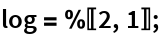In:=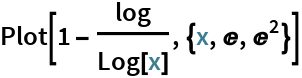Out=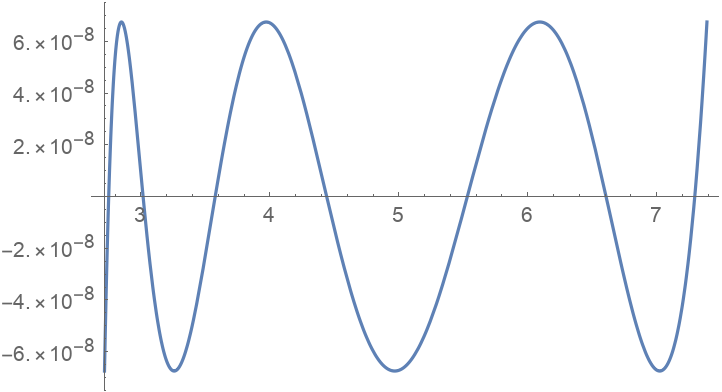Minimize the maximum absolute error:

 In:=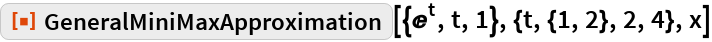Out=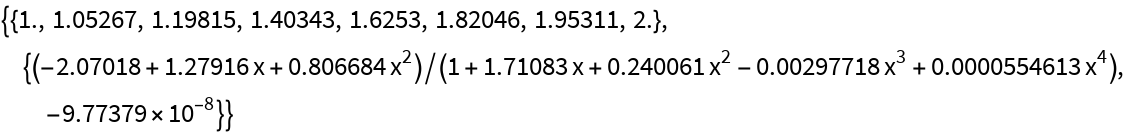The absolute error is equioscillatory:

 In:=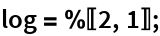In:=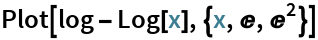Out=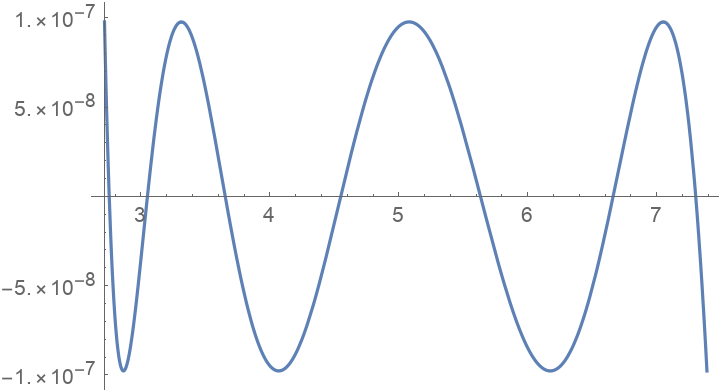### Options

#### Derivatives

A list of derivatives for both parts of a parametrically defined function, evaluated at numeric points:

 In:=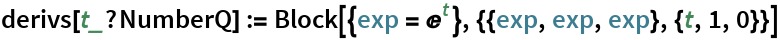The approximation:

 In:=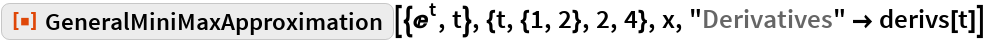Out=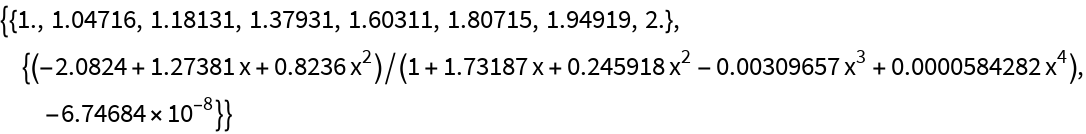### Properties and Relations

For functions with an easily computable inverse, the result given by GeneralMiniMaxApproximation is close to the one returned by MiniMaxApproximation, up to the abscissas of the extrema:

 In:=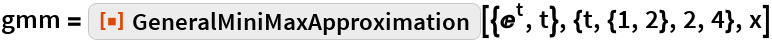Out=In:=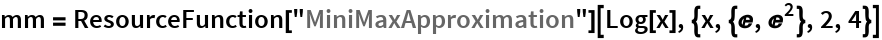Out=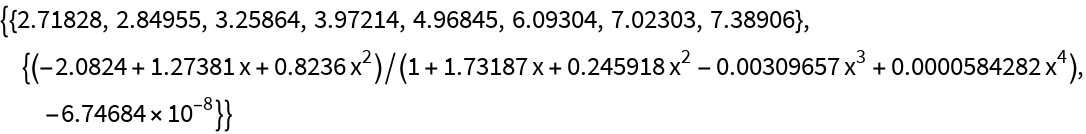In:=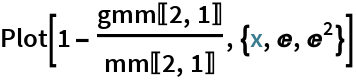Out=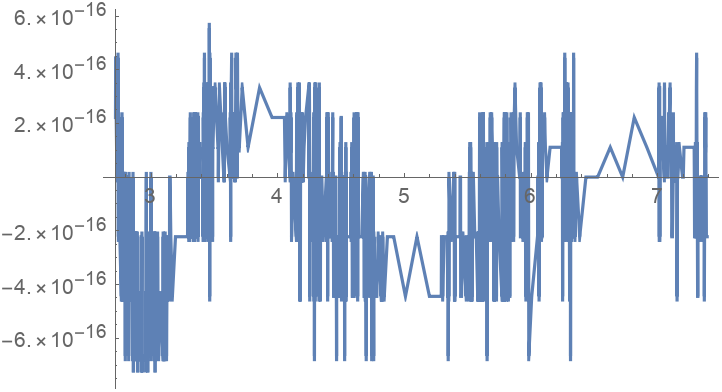## Requirements

Wolfram Language 11.3 (March 2018) or above